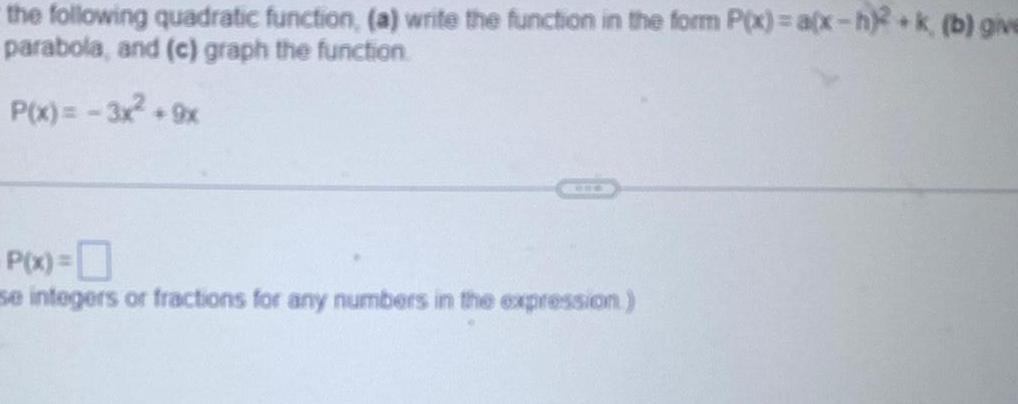Question:

# the following quadratic function a write the function in the

Last updated: 9/20/2023the following quadratic function a write the function in the form P x a x h 2 k b give parabola and c graph the function P x 3x 9x P x se integers or fractions for any numbers in the expression